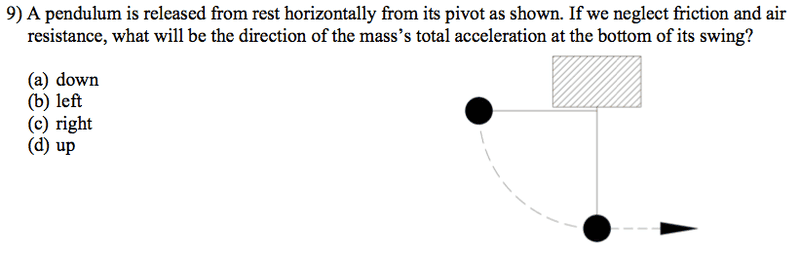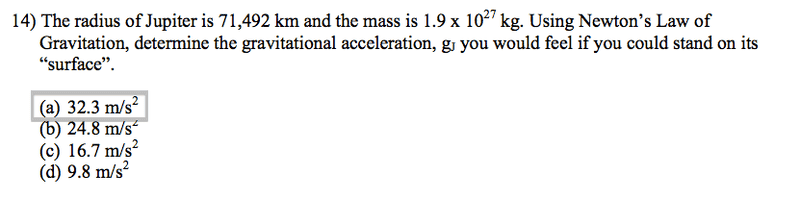# What is the mass's total acceleration at the bottom of its swing?The answer to this is up (d).
But why?
Is it because centripetal acceleration is pointing to the center of the pivot?

Also, how would I go about solving this problem :(This is what I did:

F = gm1m2/r2

FJupiter = gJupiter(m1m2/r2)
FJupiter = gJupiter(1.9 x 1027/71.4922)
FJupiter = gJupiter(3.71 x 1023)

I don't know where to go from here :(

Is it because centripetal acceleration is pointing to the center of the pivot?
I believe this is correct.

In regards to how you should go about finding the gravitational acceleration you would feel on Jupiter, keep in mind that you are supposed to be finding acceleration. You look like you were solving for the force Jupiter will exert on an object, not the acceleration of gravity on Jupiter. This is a problem because the force that Jupiter will exert on an object depends on the mass of the object. It is true that
Fg = G Mm/r2

What else is Fg equal to? Could you use that relationship to find the acceleration on Jupiter?

I believe this is correct.

In regards to how you should go about finding the gravitational acceleration you would feel on Jupiter, keep in mind that you are supposed to be finding acceleration. You look like you were solving for the force Jupiter will exert on an object, not the acceleration of gravity on Jupiter. This is a problem because the force that Jupiter will exert on an object depends on the mass of the object. It is true that
Fg = G Mm/r2

What else is Fg equal to? Could you use that relationship to find the acceleration on Jupiter?

Fg = ma

So I would do this?

mg = gjupiterm1m2/r2
(1.9 x 1027)(9.81) = gjupiter(1.9 x 1027/71.4922)
(1.9 x 1027)(9.81) = gjupiter(3.71 x 1023)
1.86 x 1028 = gjupiter(3.71 x 1023)
1.86 x 1028/(3.71 x 1023) = gjupiter
50239.89 = gjupiter
5.02 x 10-4 = gjupiter

That's wrong :(

Chestermiller
Mentor
Why did you insert gJupiter in the right hand side of the equation? G is a universal constant, and not related specifically to Jupiter. And where did the 9.81 come from? We are talking about Jupiter, not earth. In you most recent post, m is equal to m2, and g is what you are solving for; m2 is the mass of Jupiter.

Why did you insert gJupiter in the right hand side of the equation? G is a universal constant, and not related specifically to Jupiter. And where did the 9.81 come from? We are talking about Jupiter, not earth. In you most recent post, m is equal to m2, and g is what you are solving for; m2 is the mass of Jupiter.

So,
mg = (6.67 x 10-11)m1(1.9 x 1027)/71.4922
(1.9 x 1027)g = (6.67 x 10-11)m1(1.9 x 1027)/71.4922

Chestermiller
Mentor
So,
mg = (6.67 x 10-11)m1(1.9 x 1027)/71.4922
(1.9 x 1027)g = (6.67 x 10-11)m1(1.9 x 1027)/71.4922

m = m2 = your mass

m1 = mass of Jupiter

m = m2 = your mass

Just to explain this a little more to hopefully help riseofphoenix understand it: you can't plug in the mass of Jupiter to find the force Jupiter exerts on the object. Doing so would be like trying to find my weight (also known as the force that the Earth exerts on me) with the equation
w = mearth*g
That doesn't really make sense, does it? Hopefully not! If I want to find my weight, I need to use my mass! Thus to find the force that Jupiter exerts on the object, you have to multiply the mass of the object by the gravitational acceleration on Jupiter, which is why the mass of the object appears on the left side of your equation. Then you can see that the mass of the object cancels out since it is on both sides of the equation and you are left with an equation containing only known variables.

m = m2 = your mass

m1 = mass of Jupiter

This is what i did:

[STRIKE]m2[/STRIKE]g = (6.67 x 10-11)(1.9 x 1027)[STRIKE](m2)[/STRIKE]/71.4922
g = (6.67 x 10-11)(1.9 x 1027)/71.4922
g = (1.2673 x 1017)/5111.106064
g = 2.479 x 1013

???

Last edited:
The method is correct. Now you just need to check your units to make sure that they match up with the units given in the multiple choice answers.

[STRIKE]m2[/STRIKE]g = (6.67 x 10-11)(1.9 x 1027)[STRIKE](m2)[/STRIKE]/71.4922
g = (6.67 x 10-11)(1.9 x 1027)/714920002
g = (1.2673 x 1017)/(5.111106064 x 1015)
g = 24.79 m/s2

Thanks!